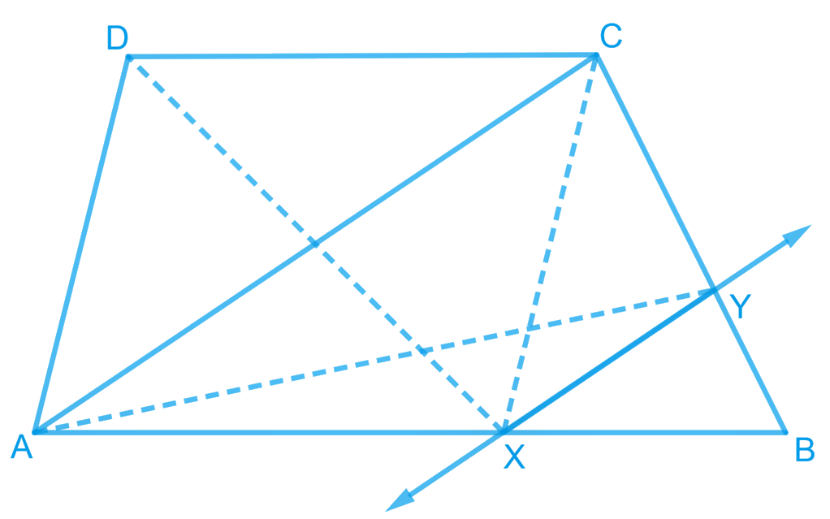# Ex.9.3 Q13 Areas of Parallelograms and Triangles Solution - NCERT Maths Class 9

## Question

$$ABCD$$ is a trapezium with $$AB\; || \;DC$$. A line parallel to $$AC$$ intersects $$AB$$ at $$X$$ and $$BC$$ at $$Y$$.

Prove that $$ar (ADX) = ar (ACY)$$.

[Hint: Join $$CX$$.]

Video Solution
Areas Of Parallelograms And Triangles
Ex 9.3 | Question 13

## Text Solution

What is known?

$$ABCD$$ is a trapezium with $$AB || DC$$. A line parallel to $$AC$$ intersects $$AB$$ at $$X$$ and $$BC$$ at $$Y.$$

What is unknown?

How we can prove that $$ar (ADX) = ar (ACY).$$

Reasoning:

We can use theorem for triangles $$ADX$$ and $$ACX$$, if two triangles are on same base and between same pair of parallel lines then both will have equal area. Similarly for triangles we can use for triangles $$ACX$$ and $$ACY$$. Now by comparing both the results, we will get the required result.

Steps:It can be observed that \begin{align}\Delta ADX \, \rm {and} \,\Delta ACX \end{align} lie on the same base $$AX$$ and are between the same parallels $$AB$$ and $$DC$$.

According to Theorem 9.2 : Two triangles on the same base (or equal bases) and between the same parallels are equal in area.

\begin{align}\therefore \text {ar }(\Delta {ADX})\!=\!\text {ar}(\Delta {ACX})\dots{(1)}\end{align}

$$\Delta ACY$$ and $$\Delta ACX$$ lie on the same base $$AC$$ and are between the same parallels $$AC$$ and $$XY$$.

According to Theorem 9.2: Two triangles on the same base (or equal bases) and between the same parallels are equal in area.

\begin{align}(\Delta {ACY})\!=\!\text {ar}({ACX})\,\ldots \text (2)\end{align}

From Equations ($$1$$) and ($$2$$), we obtain

\begin{align}{\rm{ar}}\left( {{{\Delta ADX}}} \right)\!=\!{\rm{ar}}\left( {{{\Delta ACY}}} \right)\end{align}

Video Solution
Areas Of Parallelograms And Triangles
Ex 9.3 | Question 13
Learn from the best math teachers and top your exams

• Live one on one classroom and doubt clearing
• Practice worksheets in and after class for conceptual clarity
• Personalized curriculum to keep up with school